Refer to our Texas Go Math Grade 2 Answer Key Pdf to score good marks in the exams. Test yourself by practicing the problems from Texas Go Math Grade 2 Module 2 Assessment Answer Key.

Concepts and Skills

Read the number and draw a quick picture. Then write the number in different ways. (TEKS 2.2.8, 2.2.A)

Question 1.
two hundred sixty-four
____________ hundreds __________ tens ____________ones
____________ + ____________+ ____________
____________
Answer: 2 hundreds 6 tens 4 ones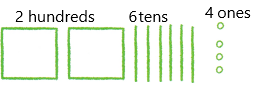Explanation:
From the above-given diagram, we can write the number in different ways.
There are four ways we can represent a number. They are:
1. Standard form
2. Expanded form
3. Word form
4. Place value chart.
1. Standard form: This is a way to write a number showing only its digits. This is the form we are most familiar with and see most often.
The number can be written in standard form as:264
2. Expanded form: This is a way to write a number as the sum of the value of its digits. This form indicates the place value of each digit in the number.
The expanded form of a number 264: 200+60+4
3. word form: This is a way to write a number in words only, also need to include commas separating the periods. If there are commas in standard form, there must be commas in word form.
The word form of 264: two hundred sixty-four
4. Place value chart: Place value in Maths describes the position or place of a digit in a number. Each digit has a place in a number. When we represent the number in general form, the position of each digit will be expanded. Those positions start from a unit place or we also call it one’s position. The order of place value of digits of a number of right to left is units, tens, hundreds, thousands, ten thousand, a hundred thousand, and so on.
The place value chart of 536: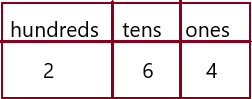2 is in the hundreds place
6 is in the tens place
4 is in the one’s place.
In Mathematics, place value charts help us to make sure that the digits are in the correct places. To identify the positional values of numbers accurately, first, write the digits in the place value chart and then write the numbers in the usual and the standard form.

Compare the numbers. Write is greater than, is less than, or is equal to. Write >, <, or =. (TEKS 2.2.D)

Question 2.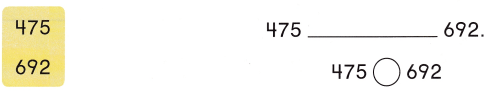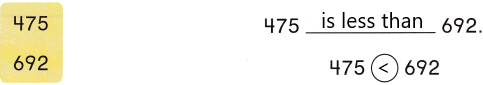Explanation:
475, 692 both the numbers have an equal number of digits, therefore, we will compare the left-most digit of both the numbers.
4 < 6
Therefore,
475 < 692
hence, 475 is smaller than 692.

Question 3.
Write numbers for the points on the number line. (TEKS 2.2.F)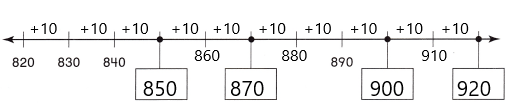Explanation:
1. Here is a number line between 820 and 920.
2. There are 10 lines dividing the total distance into 10 equal parts.
3. One whole is divided into 10 equal parts.
4. At each new line we are adding 1.
5. The digit after the point increase by 1 each time.
6. Then we have 810, 811, 812, 813, 814, 815, 816, 817, 818, 819, 820 and so on… continues up to 920.
7. Here we counting by 10’s it means we need to add 10 to the 610 then we get: 800+10=810
810+10=820
820+10=830
830+10=840
840+10=850 and so on…
8. Now write the given boxes by adding 10 to every number each time.

Question 4.
Draw and label points for 300, 345, and 375 on the number line. (TEKS 2.2.E)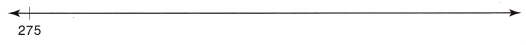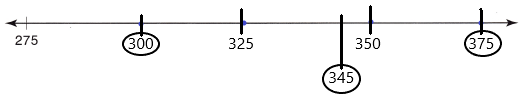Explanation:
1. Here is a number line between 275 and 375.
2. Here I count by ’25s means adding 25 to the 275 and continuing adding to the next number.
275+25=300
300+25=325
325+25=350
350+25=375
3. The above-given numbers need to plot on the number line. those are 137 and 240
4. I represented 275, 300, 325, 350, 375 on the number line and choose the intervals between the numbers. Mapped the points and highlighted them with circles.

Fill in the bubble for the correct answer choice.

Question 5.
At three schools, there are 278 students, 314- students, and 259 students. Which of these shows the numbers ordered from least to greatest? (TEKS 2.2.D)
(A) 278 < 259 < 314
(B) 259 < 278 < 314
(C) 314 < 259 < 278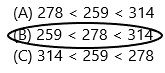Explanation:
Explanation:
1. Since, all the numbers, 259, 278 and 314 are having three digits, thus we will compare the left-most digit of the given numbers.
251 and 278 are having the same digits in hundreds value i.e., 2.
So check with another number 278 and 314
2 < 3
278 is less than 314.
Now check the numbers 259 and 278. So, we need to check the second left-most digit.
5 < 7
therefore,
259 < 278
Hence, 259 < 278 < 314.
259 is smaller than 278
278 is smaller than 314.

Question 6.
Jim used 627 craft sticks for his model boat. Which is another way to write this number? (TEKS 2.2.B)
(A) 600 + 20 + 7
(B) six twenty-seven
(C) 600 + 200 + 700Explanation:
The standard form is given:627
The above-given options are given in the expanded form.
In mathematics, the expanded form of a number is defined as the notation. It is the way of writing the numbers to define the individual digit value. When the numbers are written using place value, it will create a new algebraic expression.
It can be written as:600+20+7

Question 7.
The Sports Shop has 325 baseballs, 24-5 footballs, and 387 soccer balls. Which of these is the greatest number? (TEKS 2.2.D)
(A) 325
(B) 245
(C) 387Explanation:
The number of baseballs=325
The number of footballs=245
The number of soccer balls=387
Now compare the numbers 245, 325, and 387
1. Since, all the numbers, 245, 325 and 387 are having three digits, thus we will compare the left-most digit of the given numbers.
325 and 387 are having the same digits in hundreds value i.e., 3.
So check with another number 325 and 245
3 > 2
325 is greater than 245.
Now check the numbers 325 and 387. So, we need to check the second left-most digit.
8 < 2
therefore,
387 > 325
Hence, 387 > 325 > 245
387 is greater than other two numbers.

Question 8.
Ava wrote a number with a 3 in the tens place. Which of these could be the number? (TEKS 2.2.B)
(A) 300 + 20 + 5
(B) two hundred thirty
(C) four hundred three